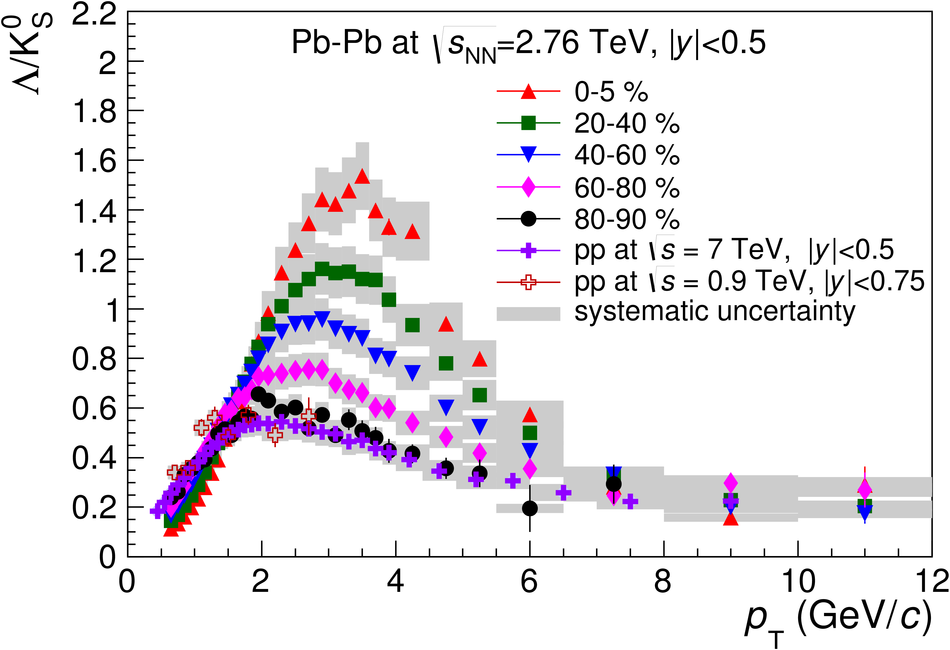# K$^0_{\rm S}$ and $\rm Λ$ production in Pb-Pb collisions at $\sqrt{s_{\rm NN}}$ = 2.76 TeV

The ALICE measurement of K$^0_{\rm S}$ and $\rm\Lambda$ production at mid-rapidity in Pb-Pb collisions at $\sqrt{s_{\rm NN}} = 2.76$ TeV is presented. The transverse momentum ($p_{\rm T}$) spectra are shown for several collision centrality intervals and in the $p_{\rm T}$ range from 0.4 GeV/$c$ (0.6 GeV/$c$ for $\rm\Lambda$) to 12 GeV/$c$. The $p_{\rm T}$ dependence of the $\rm \Lambda$/K$^0_{\rm S}$ ratios exhibits maxima in the vicinity of 3 GeV/$c$, and the positions of the maxima shift towards higher $p_{\rm T}$ with increasing collision centrality. The magnitude of these maxima increases by almost a factor of three between most peripheral and most central Pb-Pb collisions. This baryon excess at intermediate $p_{\rm T}$ is not observed in pp interactions at sqrt(s) = 0.9 TeV and at sqrt(s) = 7 TeV. Qualitatively, the baryon enhancement in heavy-ion collisions is expected from radial flow. However, the measured $p_{\rm T}$ spectra above 2 GeV/$c$ progressively decouple from hydrodynamical-model calculations. For higher values of $p_{\rm T}$, models that incorporate the influence of the medium on the fragmentation and hadronization processes describe qualitatively the $p_{\rm T}$ dependence of the $\rm\Lambda$/K$^0_{\rm S}$ ratio.

Figures

## Figure 1

 Examples of invariant mass distributions for $\Kzs$ and $\rm\Lambda$. The filled areas to the sides of the peaks were used to fit the background in order to estimate the background level under the peaks, indicated as the light shaded areas.## Figure 2

 $\Kzs$ and $\rm\Lambda$ transverse momentum spectra for different event centrality intervals shown in logarithmic (top) and linear (bottom) scale. The curves represent results of blast-wave fits.## Figure 3

 Top: $\rm\Lambda/\Kzs$ ratios as a function of $\pT$ for different event centrality intervals in Pb-Pb collisions at $\snn=2.76$ TeV and pp collisions at $\sqrt{s}=0.9$ and 7 TeV. Bottom: Selected $\rm\Lambda/\Kzs$ ratios as a function of $\pT$ compared with $\rm\Lambda/\Kzs$ and $\bar{\rm\Lambda}/\Kzs$ ratios measured in Au-Au collisions at $\snn=200$ GeV. The solid, dashed and dot-dashed lines show the corresponding ratios from a hydrodynamical model, a recombination model and the EPOS model, respectively.